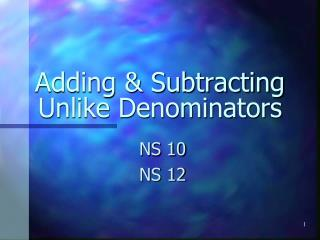DownloadDownload Presentation# Adding & Subtracting Unlike Denominators

Télécharger la présentation## Adding & Subtracting Unlike Denominators

- - - - - - - - - - - - - - - - - - - - - - - - - - - E N D - - - - - - - - - - - - - - - - - - - - - - - - - - -
##### Presentation Transcript

1. Adding & Subtracting Unlike Denominators NS 10 NS 12

2. Common Denominators The fractions ¼ and ¾ have common denominators.

3. Different Denominators The fractions ½ and ¼ do not have common denominators.

4. To add or subtract fractions that have different denominators, we first change the name of one or more of the fractions so that they have common denominators.

5. LEAST COMMON MULTIPLE The least common multiple (LCM) of the denominators is the least common denominator of the fractions.

6. Unlike denominators need to be changed before you add. Use the LCM. Find the multiples of 2 and 4: 2 2, 4, 6, 8, 10… 4 4, 8, 12, 16, 10… What is the smallest number they have in common (LCM)?

7. Since 4 is the LCM, we change the ½ so that both fractions have a denominator of 4.

9. Subtract: • We work with the fraction part of each mixed number first. • The denominators are 2 and 6. We can change halves to sixths because 6 is the LCM.

10. Then we subtract and simplify (or reduce) the answer.

11. Compare: • Rewriting fractions with common denominators can help us compare fractions. 8 is the LCM so:

12. Since then, what is the comparison? Write it on your slate.

13. Add: • For this problem we need to rename both fractions. • The denominators are 3 and 2. • What is the LCM? Write it on your slate. LCM of 3 and 2 = 6. • So the least common denominator for thirds and halves is sixths. So . . .

14. What is the LCM?Write it on your slate. 1 LCM = 9 3 1 + 9

15. What is the LCM?Write it on your slate. 1 5 LCM = 10 1 + 10

16. Add on your slate. 1 2 Having trouble? See the next slide. 1 + 8 (Don’t look if you can do it on your own.)

17. What denominator should we use? 1 2 8 x 4 = 1 + 8 x 1 = 8 Ask: What do you multiply 2 by to get 8 and what do you multiply 8 by to get 8.

18. Now multiply the numerators by the same number you multiplied the denominators. 1 x 4 = 2 8 x 4 = 1 x 1 = + 8 x 1 = 8

19. Finish by adding the like fractions. 1 4 Add only the your numerators. x 4 = 2 8 x 4 = 1 1 x 1 = + 8 x 1 = 8 5 8

20. Review: To add or subtract unlike fractions . . . • 1. Figure out what the new denominator should be. • 2. Change the name of one or both fractions. • 3. Add or subtract the fractions. • 4. Reduce the answer when possible.